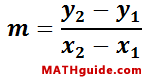Classifying Parallelograms Using Slope and Distance
View the Lesson | MATHguide homepage Updated February 2nd, 2022

Given:Quadrilateral A(-5, 9), B(1, 1), C(0, -6), D(-6, 2)Problem:Determine the slope of the diagonals and the length of the diagonals to best classify quadrilateral ABCD.

Slope of Diagonals
 mAC =mBD =Length of Diagonals
 dAC =
 dBD =

Classification: